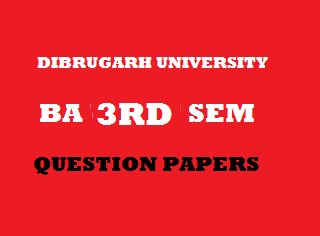## Thursday, January 03, 20192013
(November)
EDUCATION
(Major)
Course: 302
(Educational Measurement and Evaluation)
Full Marks: 80
Pass Marks: 32
Time: 3 hours
The figures in the margin indicate full marks for the questions

1. Choose the correct option/Answer the following in brief: 1x5=5
1. Which of the following is an example of measurement?
2. Kohli scored 50 in a cricket match.
3. Binod scored 65 in English.
4. All of the above.
1. Mention one of the internal factors that affect reliability of a test.
2. Write the formula to determine median from grouped data.
3. What is the number of items in the Stanford Revision of the Binet-Simon Intelligence Scale, 1916?
4. Mention one advantage of frequency polygon over histogram.
2. Fill in the blanks: 1x3=3
1. When the number of items in a test is increased, its validity ____.
2. The name of Karl Person is associated with the ____ method of determining coefficient of correlation.
3. The full form of TAT is ____.
3. Write short notes on the following: 4x4=16
1. Distinction between measurement and evaluation.
2. Blueprint of an achievement test.
3. Specific aptitude tests.
4. Scope of educational statistics.
4. What is meant by evaluation in education? Explain the terms continuous and comprehensive evaluations. 3+4+4=11
5. What is meant by a test? Describe in brief the qualities of a good test. 2+8=10
Or
Define norm. Explain the different types of norms. 2+8=10
6. Write the importance of measurement of intelligence. Distinguish between individual and group intelligence tests.        4+6=10
Or
What are self-report inventories? Describe the advantages and disadvantages of self-report inventories as tools of assessment of personality. 4+3+3=10
7. Explain the concepts of positive and negative correlations with appropriate examples. 2+2=4
8. What is meant by skewness? Explain with a diagram. 3
9. Mention two merits and two demerits of mean as a measure of central tendency. 2+2=4
10. Find out the standard deviation for the following frequency distribution table:
 Class interval Frequency 45-4940-4435-3930-3425-2920-24 3461584 N = 40

11. Find out the coefficient of correlation by rank-difference method from the following data and interpret it:   6+1=7
 Roll No.: 1 2 3 4 5 6 7 8 9 10 X: 63 70 51 85 70 45 60 65 50 55 Y: 65 70 57 75 65 55 65 73 59 45

***